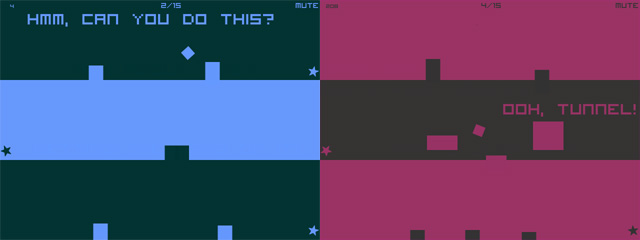# Create an HTML5 game like Space is Key with a lot of room for customization

Last month I showed you a quick but fully customizable Flash prototype of the addicting game Space is Key by Christopher Jeffrey, you can see it in the post “Create a Flash game like Space is Key with a lot of room for customization“.Now it’s time to see how to achieve the same result with HTML5, using Phaser framework.

This is what you are about to create:

Click on the stage to jump.

And this is the source code of the page. I tried to keep the same order and the same comments I used in the AS3 version

```<!doctype html>
<html>
<script src="phaser.min.js"></script>
<style>
body{margin:0}
</style>
<script type="text/javascript">
// here we define a new 640x480 game, with three core functions:
// onCreate to be executed once the game is firstly created
// onUpdate to be called every time the game us updated
// the square!! the hero of the game
var theSquare;
// square's horizontal speed in pixels/frame
var xSpeed=4;
// square's jump height, in pixels
var jumpHeight=40;
// square's jump width, in pixels. xSpeed must divide jumpWidth
var jumpWidth=120;
// rotation performed at every jump, in degrees
var jumpRotation=180;
// time passed since the player started jumping, in frames
var jumpTime=0;
// is the player jumping?
var isJumping=false;
// simple degrees to radians conversion
// array containing all various floor y positions, in pixels
var floorY=Array(92,184,276,368,460);
// floor I am currently moving on
var currentFloor=0;
// floor height, in pixels
var floorHeight=20;
// x position where the level starts, in pixels
var levelStart=0;
// x position where the level ends, in pixels
var levelEnd=640;

}

// THE GAME HAS BEEN CREATED
function onCreate() {
// simply drawing all floors, as lines from levelStart to levelEnd, tickness floorHeight
floor.lineStyle(floorHeight, 0x440044, 1);
for(i=0;i<floorY.length;i++){
floor.moveTo(levelStart,floorY[i]+floorHeight/2);
floor.lineTo(levelEnd,floorY[i]+floorHeight/2);
}
theSquare.anchor.setTo(0.5,0.5);
// event listener for mouse down
}

function jump(){
// if we aren't jumping... then JUMP!!
if (! isJumping) {
jumpTime=0;
isJumping=true;
}
}

// THE GAME IS GOING TO BE UPDATED
function onUpdate() {
// temp variable to let us know if we are on an odd or even floor
var mod=currentFloor%2;
// updating square x position
theSquare.x+=xSpeed*(1-2*mod);
// if the square reached the end of the floor...
if (theSquare.x>levelEnd&&mod==0||theSquare.x<levelStart&&mod==1) {
// move onto next floor
currentFloor++;
// if we just finished the lowest floor...
if (currentFloor>floorY.length-1) {
// start the game again
currentFloor=0;
}
// even or odd?
mod=currentFloor%2
// we start on the ground
isJumping=false;
theSquare.rotation=0;
theSquare.x=levelEnd*mod+levelStart*(1-mod);
theSquare.y=floorY[currentFloor]-game.cache.getImage("square").height/2;
}
// if we are jumping...
if (isJumping) {
// calculating the number of frames we will be jumping
var jumpFrames=jumpWidth/xSpeed;
// calculating how many degrees should the square rotate at each frame
var degreesPerFrame=jumpRotation/jumpFrames*(1-2*mod);
// calculating how may radians we have to apply to sine trigonometry function to simulate player jump
// anohter frame jumping...
jumpTime++;
// updating rotation
theSquare.angle+=degreesPerFrame;
// updating y position
// if we jumped enough...
if (jumpTime==jumpFrames) {
// ... just stop jumping
isJumping=false;
theSquare.y=floorY[currentFloor]-game.cache.getImage("square").height/2;
}
}
}

function onRender(){

}
};
</script>
<body>
</body>
</html>```

As you can see, it’s very easy to port your AS3 works in HTML5 using Phaser.

214 GAME PROTOTYPES EXPLAINED WITH SOURCE CODE
// 1+2=3
// 10000000
// 2 Cars
// 2048
// Avoider
// Ballz
// Block it
// Blockage
// Bloons
// Boids
// Bombuzal
// Breakout
// Bricks
// Columns
// CubesOut
// Dots
// DROP'd
// Dudeski
// Eskiv
// Filler
// Fling
// Globe
// HookPod
// Hundreds
// InkTd
// Iromeku
// Lumines
// Magick
// MagOrMin
// Maze
// Memdot
// Nano War
// Nodes
// o:anquan
// Ononmin
// Pacco
// Phyballs
// Platform
// Poker
// Pool
// Poux
// Pudi
// qomp
// Racing
// Renju
// SameGame
// Security
// Sling
// Slingy
// Sokoban
// Splitter
// Sproing
// Stack
// Stringy
// Sudoku
// Tetris
// Threes
// Toony
// Turn
// TwinSpin
// vvvvvv
// Wordle
// Worms
// Yanga
// Zhed
// zNumbers